# Test: Fourier Analysis of Discrete Time Signals

## 10 Questions MCQ Test Topicwise Question Bank for Electronics Engineering | Test: Fourier Analysis of Discrete Time Signals

Description
Attempt Test: Fourier Analysis of Discrete Time Signals | 10 questions in 30 minutes | Mock test for GATE preparation | Free important questions MCQ to study Topicwise Question Bank for Electronics Engineering for GATE Exam | Download free PDF with solutions
QUESTION: 1

### The discrete timefourier transform for the given signal x[n] - u[n] is

Solution: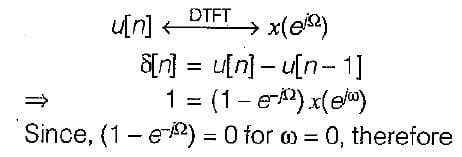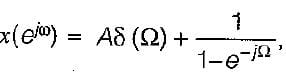where A is constant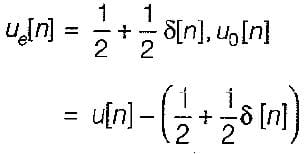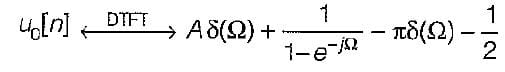The Fourier transform of odd real sequences must be purely imagenary, thus A = π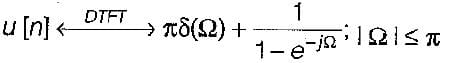QUESTION: 2

### The discrete time Fourier coefficients x[k] of the signal x [n ] =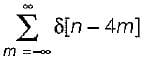Solution: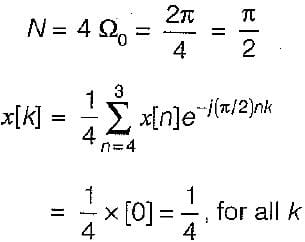QUESTION: 3

### The zero state respone y(k) for input f(k) = (0.8)k u(k) is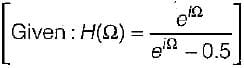Solution: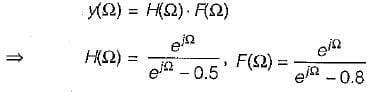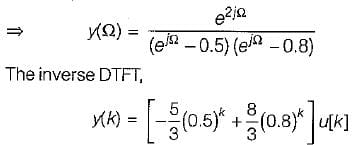QUESTION: 4

Consider discrete time sequence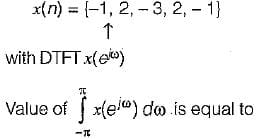Solution: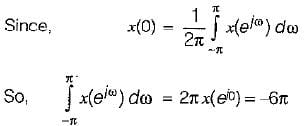QUESTION: 5

Consider a signal x(n) with following factors:
1. x(n) is real and even signal
2. The period of x(n) is N = 10
3. x(11) = 5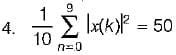The signal x(n) is

Solution: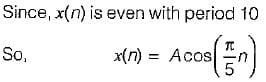QUESTION: 6

Consider a discrete time signal x(n) = {-1, 2, -3, 2, -1} value of ∠x(e) is equal to

Solution:
QUESTION: 7

A low pass filter with impulse response h1(n) has spectrum H1 (e) shown below.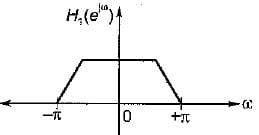Here only one period has been shown by reversing every second sign of h1(n) a new filter having impulse response  h2(t) is created. The spectrum of H2(e) is given by

Solution:
QUESTION: 8

A red signal x[n] with Fourier transform x(e) has following property:
1. x[n] = 0 for, n > 0
2. x [ 0] > 0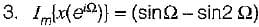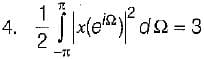The signal x[n] is

Solution:
QUESTION: 9

A causal and stable LTI system has the property that,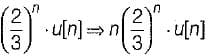The frequency response H(e) for this system is

Solution:
QUESTION: 10

A 5-point sequence x[n] is given as 4 [- 3] = 1, x[ - 2] = 1, x[ -1 ] = 0 . x = 5 , x - 1
Let x(e) denote the discrete time fourier transform of x[n].
The value of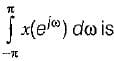Solution:Use Code STAYHOME200 and get INR 200 additional OFF Use Coupon Code